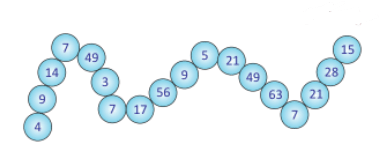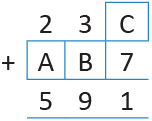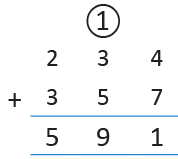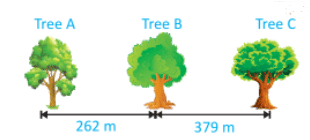Olympiad Test Level 1: Computing Operations

# Olympiad Test Level 1: Computing Operations

Test Description

## 20 Questions MCQ Test | Olympiad Test Level 1: Computing Operations

Olympiad Test Level 1: Computing Operations for Class 3 2023 is part of Class 3 preparation. The Olympiad Test Level 1: Computing Operations questions and answers have been prepared according to the Class 3 exam syllabus.The Olympiad Test Level 1: Computing Operations MCQs are made for Class 3 2023 Exam. Find important definitions, questions, notes, meanings, examples, exercises, MCQs and online tests for Olympiad Test Level 1: Computing Operations below.
Solutions of Olympiad Test Level 1: Computing Operations questions in English are available as part of our course for Class 3 & Olympiad Test Level 1: Computing Operations solutions in Hindi for Class 3 course. Download more important topics, notes, lectures and mock test series for Class 3 Exam by signing up for free. Attempt Olympiad Test Level 1: Computing Operations | 20 questions in 40 minutes | Mock test for Class 3 preparation | Free important questions MCQ to study for Class 3 Exam | Download free PDF with solutions
 1 Crore+ students have signed up on EduRev. Have you?
Olympiad Test Level 1: Computing Operations - Question 1

### Find the missing number 165 + 52 + 10 = 52 + ? + 10

Detailed Solution for Olympiad Test Level 1: Computing Operations - Question 1

According to order

property of addition, the numbers can be added in any order. So,

165 + 52 + 10 = 52 + 165 + 10

Olympiad Test Level 1: Computing Operations - Question 2

### If A = 25 + 7,825 and B = 4,525 + 75, find the difference between A and B.

Detailed Solution for Olympiad Test Level 1: Computing Operations - Question 2

A = 25 + 7,825 = 7,850

B = 4,525 + 75 = 4,600

A – B = 7,850 – 4,600 = 3,250

Olympiad Test Level 1: Computing Operations - Question 3

### Look at the two number sentences shown below :8 × = 40, 40 ÷ 8 =Which number goes in each box to make each number sentence true ?

Detailed Solution for Olympiad Test Level 1: Computing Operations - Question 3

8 × 5 = 40 and 40 ÷ 8 = 5

Olympiad Test Level 1: Computing Operations - Question 4

Simplify : 100 – 10 – (100 – 10)

Detailed Solution for Olympiad Test Level 1: Computing Operations - Question 4

(100 – 10) – (100 – 10)= 90 – 90 = 0

Olympiad Test Level 1: Computing Operations - Question 5

The sum of 1,727 and 6,475 is ________.

Detailed Solution for Olympiad Test Level 1: Computing Operations - Question 5

1,727 + 6,475 = 8,202

Olympiad Test Level 1: Computing Operations - Question 6

347 × 23 = ____________

Detailed Solution for Olympiad Test Level 1: Computing Operations - Question 6

The product of one’s digits, that is, 3×7 = 21. So, the one digit of the product will be 1. The

product of 347 and 23 is 7,981.

Olympiad Test Level 1: Computing Operations - Question 7

112 is divisible by which one of the following numbers ?

Detailed Solution for Olympiad Test Level 1: Computing Operations - Question 7

Last digit is 2, so it is an even number. Thus 112 is divisible by 2.

Olympiad Test Level 1: Computing Operations - Question 8

Simplify: 4 + 14 + 1

Detailed Solution for Olympiad Test Level 1: Computing Operations - Question 8

4 + 14 + 1 = 18 + 1 = 19

Olympiad Test Level 1: Computing Operations - Question 9

How many numbers given in the figure come in the table of 7 ?Detailed Solution for Olympiad Test Level 1: Computing Operations - Question 9 The numbers divisible by 7 are 14, 7, 49, 7, 56, 21, 49, 63, 7, 21 and 28.
Olympiad Test Level 1: Computing Operations - Question 10

Which of the following represents the additive identity property of 0 ?

Detailed Solution for Olympiad Test Level 1: Computing Operations - Question 10

It means that additive identity is “0” as adding 0 to any number, gives the sum as the number itself. For any set of numbers, that is, all integers, rational numbers, complex numbers, the additive identity is 0.

So here, 14 + 0 = 14 is the correct answer.

Olympiad Test Level 1: Computing Operations - Question 11

Difference between the two numbers is 400. If one of the numbers is 300 greater than 600, find the other number.

Detailed Solution for Olympiad Test Level 1: Computing Operations - Question 11

One number is 300 greater than 600 = 600 + 300 = 900 Difference = 400

Other number = 900 – 400 = 500

Olympiad Test Level 1: Computing Operations - Question 12

Find the missing number to fill in the blank box

111 + 9 = ⌧+ 7

Detailed Solution for Olympiad Test Level 1: Computing Operations - Question 12

111 + 9 = 120 = 113 +7

Olympiad Test Level 1: Computing Operations - Question 13

How many 7s are there in 63 ?

Detailed Solution for Olympiad Test Level 1: Computing Operations - Question 13

63 ÷ 7 = 9

Olympiad Test Level 1: Computing Operations - Question 14

Find the value of A, B and C respectively.Detailed Solution for Olympiad Test Level 1: Computing Operations - Question 14Olympiad Test Level 1: Computing Operations - Question 15

In an examination hall, 500 chairs were arranged equally in 10 rows. How many chairs are there in each row ?

Detailed Solution for Olympiad Test Level 1: Computing Operations - Question 15

Number of chairs in each row = 500 ÷ 10 = 50

Olympiad Test Level 1: Computing Operations - Question 16

Which of the following is true ?

Detailed Solution for Olympiad Test Level 1: Computing Operations - Question 16

43 is more than 33.

Olympiad Test Level 1: Computing Operations - Question 17

Add the 6,523 + 2,364. Which one of the following numbers is in the hundreds place of the resulting addition ?

Detailed Solution for Olympiad Test Level 1: Computing Operations - Question 17

6523 + 2364 = 8887

So, digit 8 is hundreds of places.

Olympiad Test Level 1: Computing Operations - Question 18

When we divide 0 by 3, we get _______.

Detailed Solution for Olympiad Test Level 1: Computing Operations - Question 18

0 divided by any number gives 0.

So, 0 ÷ 3 = 0.

Olympiad Test Level 1: Computing Operations - Question 19

I am the smallest odd number that comes after 4,386. Add 632 to me. What number am I now ?

Detailed Solution for Olympiad Test Level 1: Computing Operations - Question 19

Smallest odd number after 4,386 is 4,387.

4,387 + 632 = 5,019

Olympiad Test Level 1: Computing Operations - Question 20

Sandhya cycled from Tree B to Tree C. She then cycled from Tree C to Tree A. How far did she cycle altogether ?Detailed Solution for Olympiad Test Level 1: Computing Operations - Question 20

A = 25 + 7,825 = 7,850

B = 4,525 + 75 = 4,600

A – B = 7,850 – 4,600 = 3,250(Scan QR code)